Formulas for Schools Students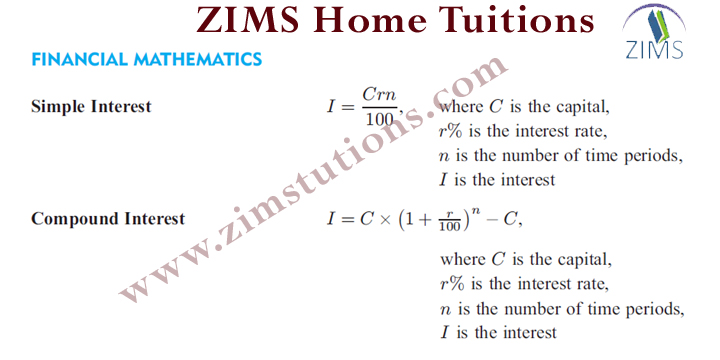Mathematics for the international student.Download formulas to make things hand and easy to solve complex problems.

Formulas of Calculas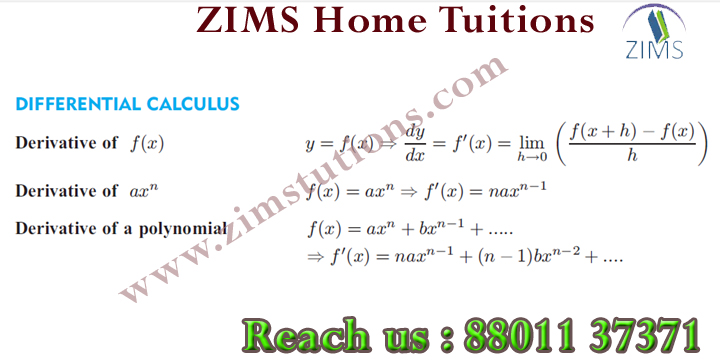Make things more Easy.
It is possible with zims academy.
www.zimstutions.com

Formulas on Probability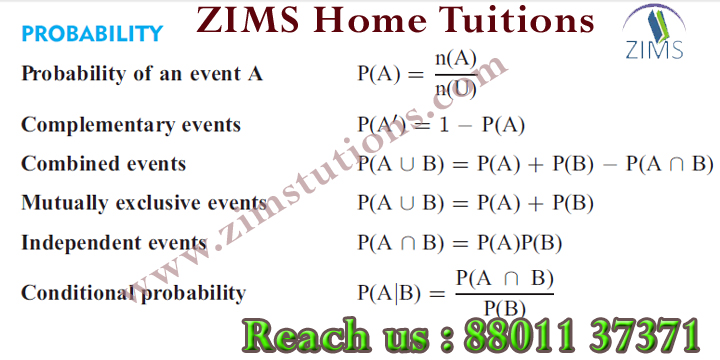Make thing logical only at
www.zimstutions.com

Formulas of Finite numbers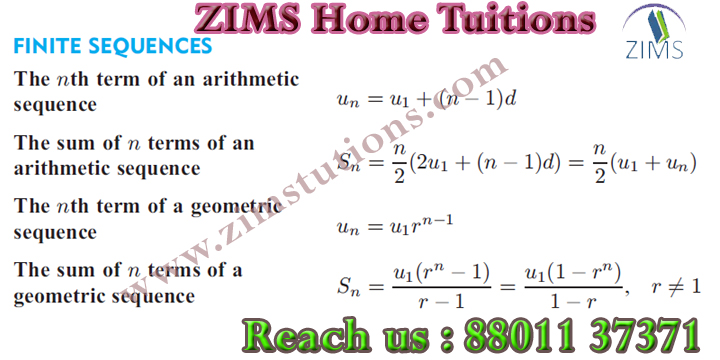Formulas of Finite numbers.
Make complex problem easy only at zims
call us on +91 88011 37371
MENSURATION Formulas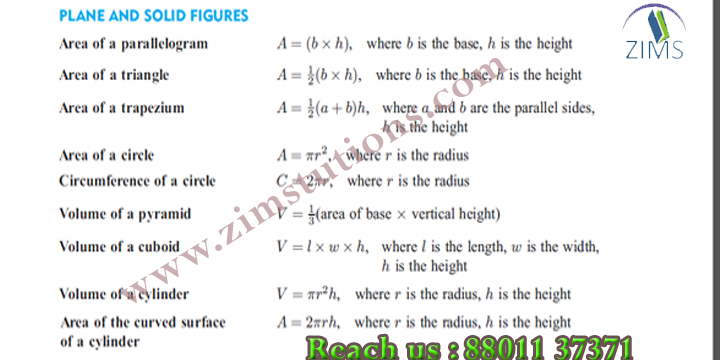Mensuration is the branch of mathematics which deals with the study of Geometric shapes, their area, volume and related parameters.

Some important mensuration formulas are:

1. Area of rectangle (A) = length(l) × Breath(b)

2. Perimeter of a rectangle (P) = 2 × (Length(l) + Breath(b))

3. Area of a square (A) = Length (l) × Length (l)

MENSURATION Formulas-2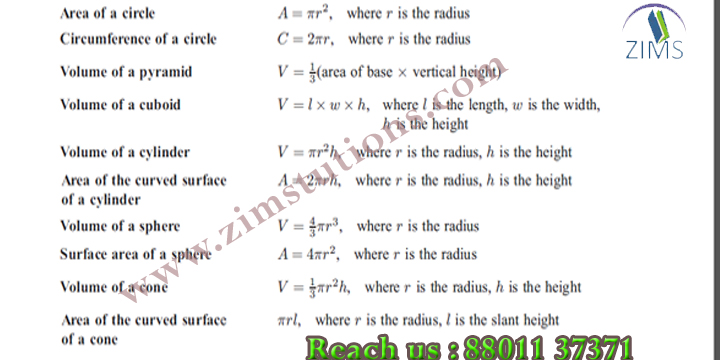Mensuration is the branch of mathematics which deals with the study of Geometric shapes, their area, volume and related parameters.

Area of isosceles triangle =$\frac{b}{4}\sqrt{4a^2 - b^2}$

Where a = length of two equal side , b= length of base of isosceles triangle.

Area of trapezium (A) =$\frac{1}{2} (a+b) \times h$

Where “a” and “b” are the length of parallel sides and “h” is the perpendicular distance between “a” and “b” .

Perimeter of a trapezium (P) = sum of all sides

Area of rhombus (A) =  Product of diagonals / 2

Algebraic expression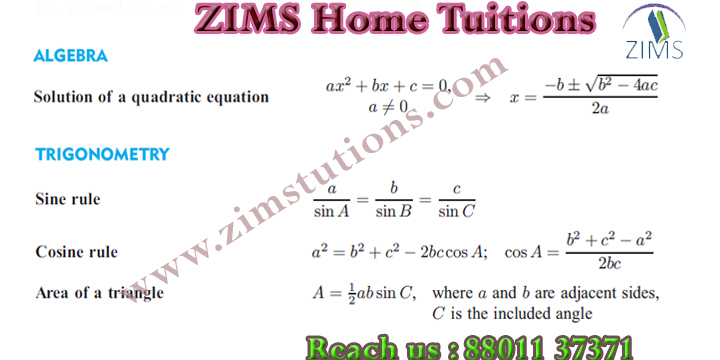In mathematics, an algebraic expression is an expression built up from constants, variables, and a finite number of algebraic operations. For example, is an algebraic expression.

Get more of Maths knowledge
Geometric Formulas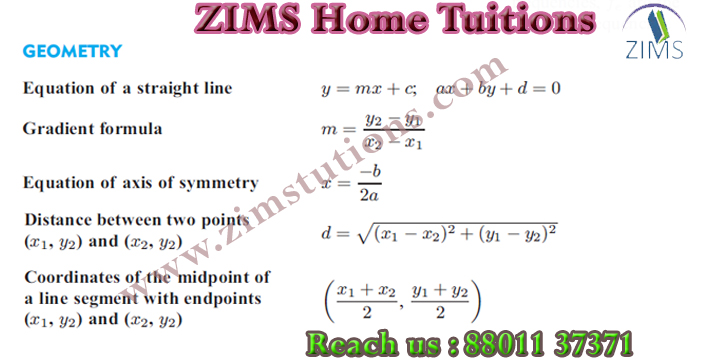In mathematics, the geometric mean is a type of mean or average, which indicates the central tendency or typical value of a set of numbers by using the product of their values.

This is calculated by multiplying all the numbers (call the number of numbers n), and taking the nth root of the total.
Statistical Formulas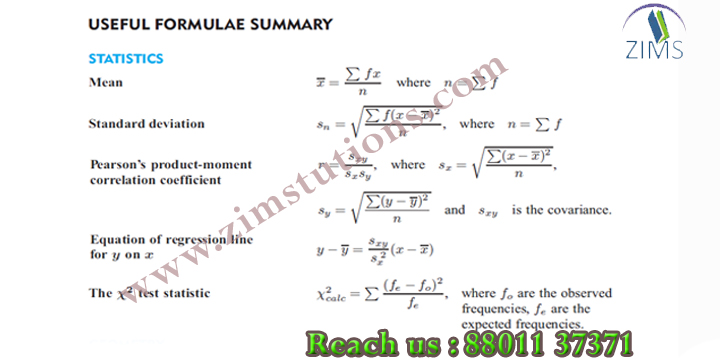One of the most basic purposes of statistics is simply to enable us to make sense of large numbers. For example, if you want to know how the students in your school are doing in the statewide achievement test, and somebody gives you a list of all 600 of their scores, that’s useless. This everyday problem is even more obvious and staggering when you’re dealing, let’s say, with the population data for the nation.

We've got to be able to consolidate and synthesize large numbers to reveal their collective characteristics and interrelationships, and transform them from an incomprehensible mass to a set of useful and enlightening indicators.

Mathematical symbols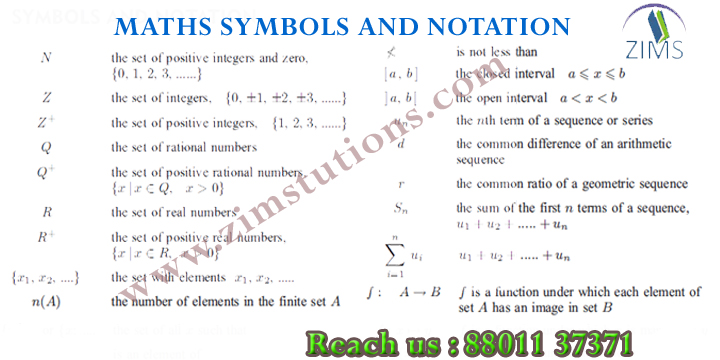# Mathematical Symbols

Mathematical symbols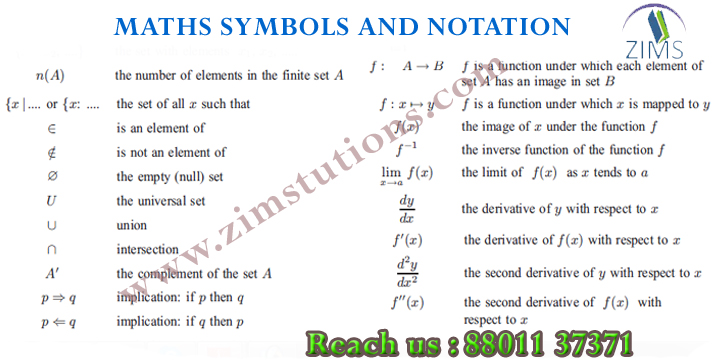Mathematical symbols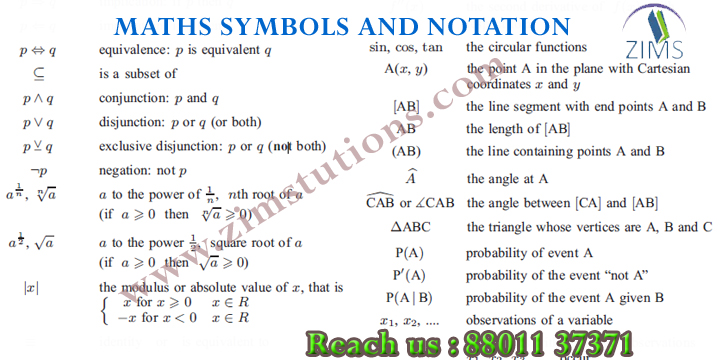maths symbols
maths formula